# CSS Functions| Part 1

## Introduction

Surfing through the web is as easy as clicking a button.

Source: giphy

But have you ever wondered about the trouble behind putting up a website?

What if you opened Facebook and the page looked like this:

Pretty boring, huh?

That’s how important it is to style a webpage.

The primary markup language used to style web pages is CSS (cascading style sheet). I’m pretty sure you already knew that, but did you know that, like any other programming language, there are functions in CSS too?

To formally define them,

CSS functions are predefined functions in CSS that enhance the presentation of web pages by altering colours, layout, and fonts.

## Difference Between CSS Functions and Normal Functions

Before moving on to the actual CSS functions, let us see some basic differences between functions in CSS and the functions we usually use in any programming language.

In any other programming language, functions may be predefined or user-defined. In contrast to this, CSS functions are only predefined. A concept similar to user-defined functions can, however, be implemented using CSS selectors.

Apart from this, in any programming language, the result of functions are usually returned and then used elsewhere in the program.

For example, in the Python code below, the function returns the sum of two numbers, which is used to find the average numbers in a list.

Output:

In CSS functions, an output is produced, which is visible in the layout of the webpage.

For example, the colour of an element may be specified using the rgb( ) or rgba( ) method.

Don’t worry if the names of these functions are confusing you because next, we’ll learn about the different CSS functions.

## Types of CSS Functions with Examples

So far, we only know a bit about CSS functions, but we haven’t seen any examples.

Then what are we waiting for?

Let’s see the different types of CSS functions.

## CSS Custom Properties

Do you remember what a big advantage of using functions was?

If you’re thinking about reusability, you’re right.

The var( ) function in CSS is used for reusability too. To understand this better, let us see an example.

HTML:

CSS:

Output:

In the style sheet, two colours, red and blue, were already specified. Then, for the three paragraphs (from the HTML code), we specified the colours of each paragraph using the var( ) function.

## Colour Functions

There are two colour functions in CSS - rgb( ) and hsl( ). These two again have another variation - rgba( ) and hsla( ), where ‘a’ denotes alpha which determines the opacity of the colour. You can read more about CSS colour here.

## Pseudo Class Selector Functions

We have all seen tables where every other row is of a different colour, making the table background look striped.

Have you ever wondered how they are made?

Well, pseudo-class selector functions are the answer. There are two kinds of these functions that can be used to style a page. Let’s see what they are.

### :nth-child( )

This function takes an argument that is an equation in n to style only those elements.

The example given below styles every other paragraph.

HTML:

CSS:

Output: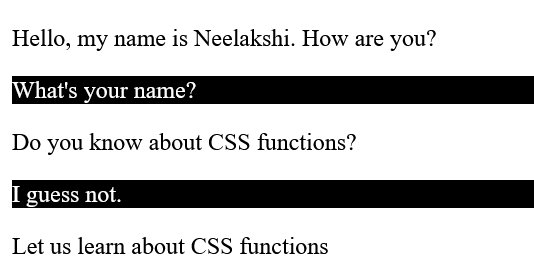### :nth - last - child( )

This method starts counting from the end and styles the elements accordingly.

For example, in the code below, every 4th element from the end is styled.

CSS:

Output: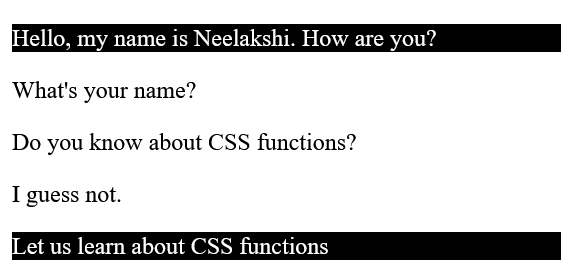### :nth - of - type( )

Here, we can specify the type of the element, for example, even or odd. We can also mention the position of the element.

#### Example 1:

CSS:

Output: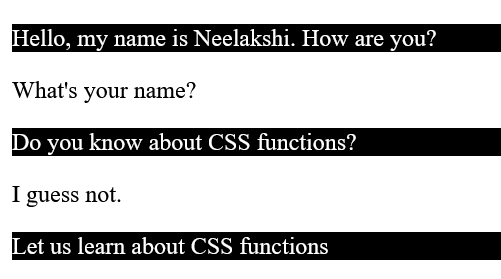#### Example 2:

CSS:

Output: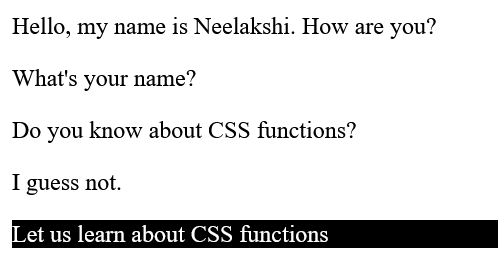### :nth - last - of - type( )

This is similar to nth-last-child, where the elements are counted from the end and styled accordingly.

For example, the 5th element from the end, i.e., the first element, will be styled only in the code below.

CSS:

Output: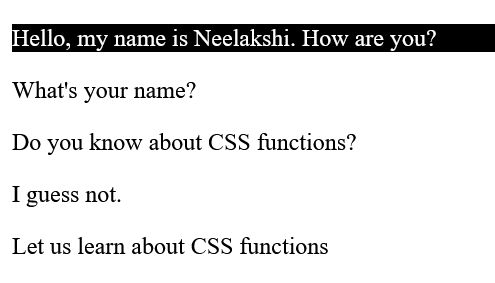## Sizing and Scaling or Transform Functions

In the introduction, we saw a picture of Facebook without any styling. None of the elements was scaled or arranged correctly on the page. So how do we scale the elements?

By using the sizing and scaling functions, of course!

Let’s see the different sizing and scaling functions in CSS.

### scaleX( ), scaleY( ), scaleZ( ), scale3d( ) and scale( )

The scaleX( ), scaleY( ), scaleZ( ), scale3d( ) and scale( ) are used to scale elements along the x-axis, y-axis, z-axis, all three axes and in a 2-d plane, respectively.

The value given as a parameter determines the fraction of scaling.

The code below shows how the scaleX( ) function scales elements along the x-axis.

CSS:

Output:

### translateX( ),translateY(), translateZ(), translate3d(), and translate()

Similar to the scale function, the translate function can translate any element along all three axes’ individually and simultaneously and in a 2-d plane.

A percentage or number of pixels determining the translation is passed as a parameter to the function.

An example of translateX( ) is shown below.

CSS:

Output:

Here, the paragraph is translated along the x-axis by 50 pixels.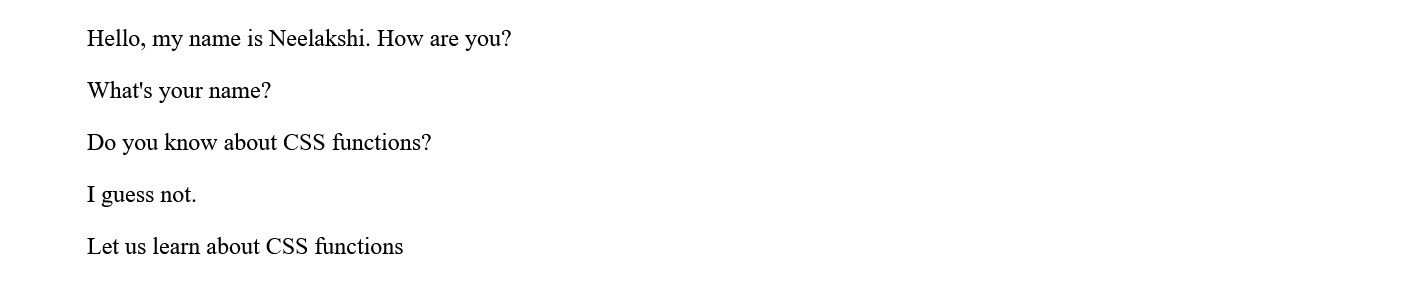### rotateX(), rotateY(), rotateZ(), rotate3d(), and rotate()

These functions are used to rotate an element along the x-axis, y-axis, z-axis, all three axes’ or in a 2-D plane.

In the example below, we rotate div2 along the x-axis to produce a 3-D effect using the perspective attribute.

HTML:

CSS:

Output: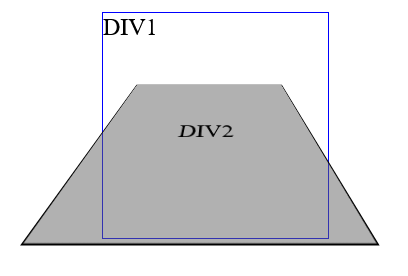### skewX( ), skewY( ) and skew( )

These methods are used to distort an element. This will be better understood with the help of an example.

CSS:

Output: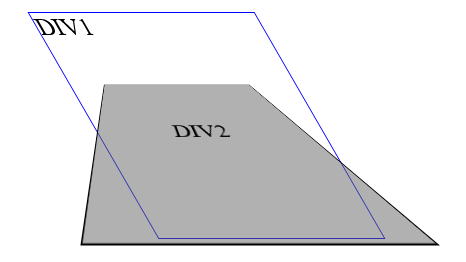1. What are CSS functions?
CSS functions are predefined functions in CSS that enhance the presentation of web pages by altering colours, layout, and fonts.

2. How are CSS functions different from regular functions?
CSS functions differ from normals functions in the following ways:
They are always predefined.
They always give an output in the form of changes in the presentation of web pages.

3. What are the advantages of CSS functions?
Functions in CSS can be easily used to style web pages. Moreover, they also facilitate reusability like normal functions.

4. Name some common CSS functions.
Some common functions in CSS are:
rgb( )
scale( )
translate( )
rotate( )
skew( )

5. What is the difference between rgb( ) and rgba( )?
The rgb( ) function requires only three parameters - the intensity of red, green and blue, respectively. In contrast, the rgba( ) function requires an additional parameter alpha that determines the opacity of an element.

## Key Takeaways

In this article, we learn what CSS functions are. We also saw some different kinds of functions in CSS and examples of them, but they are not the only functions available in CSS.

There are so many more functions about which we don’t know yet. So without further ado, let’s learn about them in the second part of this article - CSS Functions Part 2.